# Area of shape + circular sector - examples

1. Circle arcCircle segment has a circumference of 72.95 cm and 1386.14 cm2 area. Calculate the radius of the circle and size of central angle.
2. Circular poolThe base of pool is circle with a radius r = 10 m excluding circular segment that determines chord length 10 meters. Pool depth is h = 2m. How many hectoliters of water can fit into the pool?
3. Arc and segmentCalculate the length of circular arc l, area of the circular arc S1 and area of circular segment S2. Radius of the circle is 33 and corresponding angle is ?.
4. Goat and circlesWhat is the radius of a circle centered on the other circle and the intersection of the two circles is equal to half the area of the first circle? This task is the mathematical expression of the role of agriculture. The farmer has circular land on which g
5. Circle and angleWhat is the length of the arc of a circle with radius r = 207 mm with cental angle 5.33 rad?
6. Park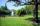In the newly built park will be permanently placed a rotating sprayer irrigation of lawns. Determine the largest radius of the circle which can irrigate by sprayer P so not to spray park visitors on line AB. Distance AB = 55 m, AP = 36 m and BP = 28 m.
7. Circle arcCalculate the area of the circular arc in m2 where the diameter is 290 dm and a central angle is 135°. Result round to three decimal places.
8. Circle sectorCircular sector with a central angle 80 ° has area 1257 cm2. Calculate its radius r.
9. Field with vegetables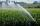Field planted with vegetables has shape of a rectangular isosceles triangle with leg length of 24 m. At the vertices of the triangle are positioned rotating sprinklers with a range of 12 m. How much of the field sprinkler doesn't irrigated?
10. Equilateral triangle v3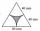Calculate the content of the colored gray part. Equilateral triangle has side length 8 cm. Arc centers are the vertices of a triangle.
11. Math heart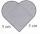Stylized heart shape created from a square with side 5 cm and two semicircles over his sides. Calculate the content area and its circumference.
12. Cylinder horizontallyThe cylinder with a diameter of 3 m and a height/length of 15 m is laid horizontally. Water is poured into it, reaching a height of 60 cm below the axis of the cylinder. How many hectoliters of water is in the cylinder?
13. Irrigation sprinklerThe irrigation sprinkler can twist with an angle of 320° and has a reach of 12 meters. Which area can irrigate?
14. Three segments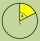The circle is divided into 3 segments. Segment A occupies 1/4 of the area, segment B occupies 1/3 of the area. What part is occupied by section C? In what proportion are areas A: B: C?

We apologize, but in this category are not a lot of examples.
Do you have an interesting mathematical example that you can't solve it? Enter it, and we can try to solve it.

To this e-mail address, we will reply solution; solved examples are also published here. Please enter e-mail correctly and check whether you don't have a full mailbox.